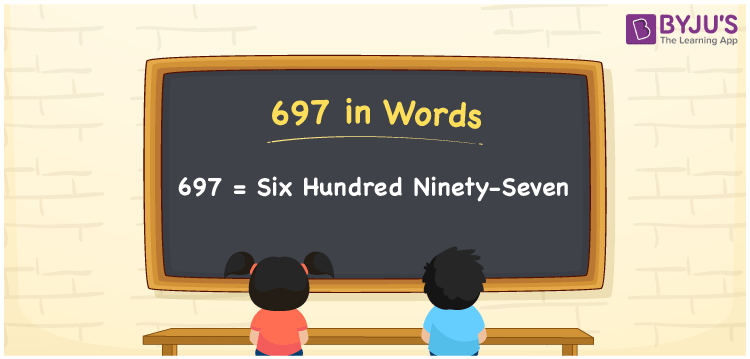# 697 in Words

697 in words is written as Six hundred ninety-seven. If you paid Rs. 697 for a book, then you can say, “I paid Six hundred ninety-seven rupees for the book”. Here, Six hundred ninety-seven represents the word form or number name of 697. In this article, you will learn how to convert the cardinal number 697 into words and some facts about 697.

 697 in words Six hundred ninety-seven Six hundred ninety-seven in Numbers 697

## 697 in English Words

We generally use the English alphabet to write numbers in words. So, we spell 697 in English words as “Six hundred ninety-seven”.## How to Write 697 in Words?

Below is the place value chart for the number 697. Using the below table, we can easily derive the word form of 697.

 Hundreds Tens Ones 6 9 7

Here, ones = 7, tens = 9, hundreds = 6

By expanding the above number with respect to the place values, we get;

6 × Hundred + 9 × Ten + 7 × One

= 6 × 100 + 9 × 10 + 7 × 1

= 600 + 90 + 7

= Six hundred + Ninety + Seven

= Six hundred ninety-seven

Therefore, 697 in words is written as Six hundred ninety-seven.

As we know, 697 is a natural number that precedes 698 and succeeds 696.

697 in words – Six hundred ninety-seven

Is 697 an even number? – No

Is 697 an odd number? – Yes

Is 697 a prime number? – No

Is 697 a composite number? – Yes

Is 697 a perfect square number? – No

Is 697 a perfect cube number? – No

## Frequently Asked Questions on 697 in Words

Q1

### How do you write 697 in word form?

We can write the number 697 in word form as Six hundred ninety-seven.
Q2

### How to write Rs. 697 in words on a cheque?

On a cheque, an amount of Rs. 697 can be written in words as “Six hundred ninety-seven rupees only”.
Q3

### Write the number 697.50 in words.

The decimal number 697.50 is written in words as “Six hundred ninety-seven point five zero”.